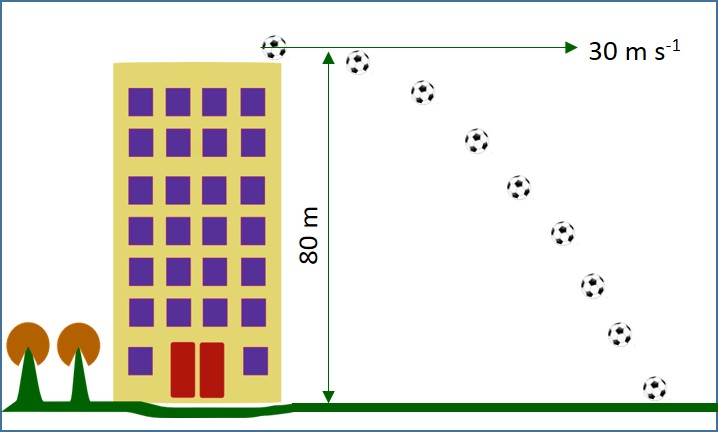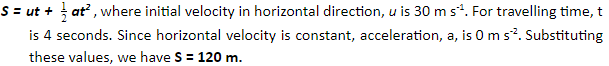# Projectile Motion

## Introduction

Consider a car that is moving in a straight line. The motion of the car is one-dimensional. Now, consider a ball kicked by a football player. The motion of the ball is two-dimensional, as illustrated in Figure 1. In this article, the concept of two-dimensional motion and the equations that govern the two-dimensional motion are explained.Figure 1: Two-dimensional Motion

A. Projectile Motion

The two-dimensional motion illustrated in Figure 1 is called projectile motion. The curve traced out by the object subject to a constant force in one direction is a parabola. The projectile motion of a particle can be studied by splitting the motion into horizontal and vertical components, and considering the forces that move the object separately. Figure 2 explains a scenario where projectile motion happens. The horizontal force acting on the object tends to move the object horizontally and the gravitational force acting on the object tends to move the object downwards. The combination of horizontal force and gravitational force tends to move the object in a parabolic path, as illustrated in the following drawing.Figure 2: Two-dimensional Motion

B. Governing Equation

If a particle projected with velocity (u) at an angle (Ө) to the horizontal from a point on level ground, the range (R) is defined as the distance from the point of projection to the point at which the particle reaches the ground again. The range (R) can be calculated using the formula:Figure 3: Projectile Motion

C. Example of Application

A ball is thrown from the top of a building, 45 m high above ground level, with an initial velocity of 15 m s-1 in a horizontal direction (Figure: 4). How long does it take to reach the ground? How far from the base of the building is it when it reaches the ground?Figure 4: Two-dimensional Motion

SolutionNow, it is known that the ball travels for 4 seconds before reaching the ground. Applying this value in horizontal component of the motion, the horizontal distance travelled can be calculated.D. Summary

• Projectile motion is the two-dimensional motion of an object due to the external force and gravity.
• Projectile motion of any object is a parabola.
• The motion of projectiles is analysed in terms of two independent motions at right angles. The horizontal component of the motion is at a constant velocity, while the vertical motion is subject to a constant acceleration due to gravity (g).
• The range (R) of an object in projectile motion is defined as the distance from the point of projection to the point at which the particle reaches the ground again.